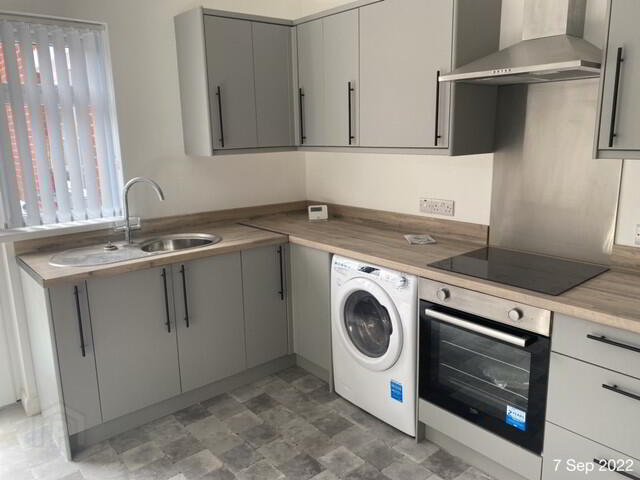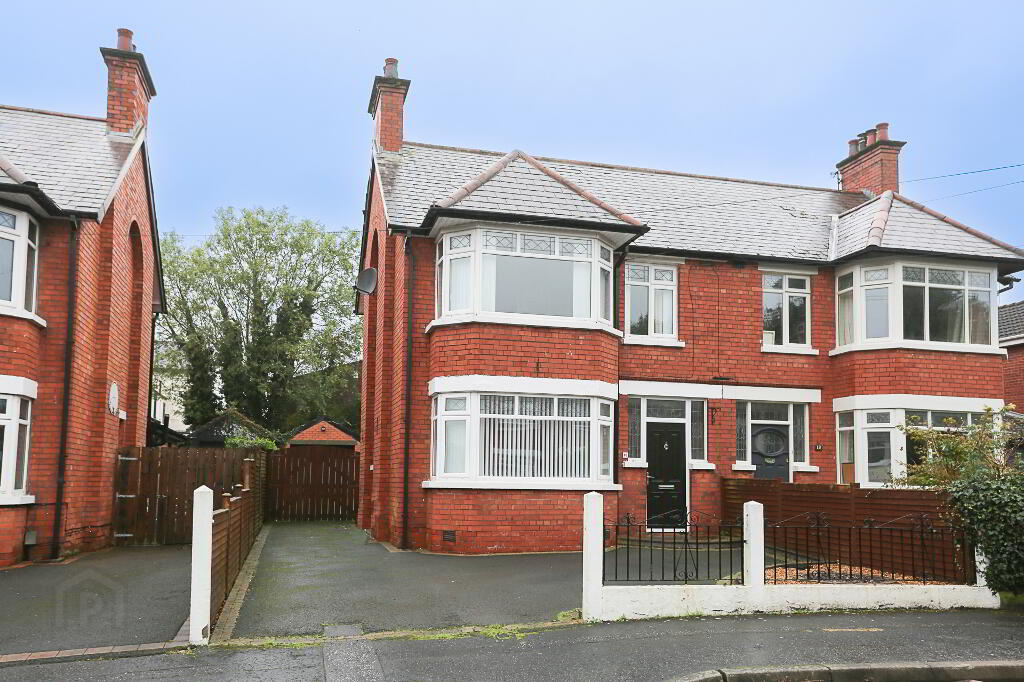Type
Sale/Rent
Min Price
Max Price
Min Beds
Max Beds

# Property For Rent Showing properties 1 to 12 of 42

Sort by
• To let£725 / month
• To let£850 / month
• To let£795 / month
• Let agreedLet agreed £1,400 / month
• Let agreedLet agreed £600 / month
• Let agreedLet agreed £1,100 / month
• LetLET
• LetLET
• Let agreedLet agreed £795 / month
• LetLET
• LetLET
• LetLET